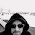## Thursday, 29 December 2011

### A different 2D array in bash

I have recently been writing a pretend Object Oriented Programming layer on top of bash. Basically, it uses some global vars, function-within-functions and a ton of eval to create the illusion of OOP in bash.

Something like:

```#!/usr/lib/oobash.sh

# Import the Car class
`import Car`

# Create a new "object"
new Car as mycar

# Set a variable
mycar.speed 100

# Call a function
mycar.drive
```

This will be made available here http://code.google.com/p/object-oriented-bash/ soon, but in the meantime I have needed to implement a 2D array in bash. A quick Google search shows some different ways of doing this. Firstly is to dynamically create arrays using eval. The other approach is to use a huge offset, e.g. 1000, then refer to array[i][j] as array[i*1000+j] etc. The latter example requires you to keep track of two indexes which is sort of annoying.

Edit:

I noticed someone has already done something similar at http://oobash.sourceforge.net/. I'l take a look at it some point and if it does the job I'll abandon my project.

So, in an attempt to find something different, I came up with the following. The idea is to store some metadata about how many elements we're adding.

An array with just one element might look like this:

``` = 1
 = "Hello there"
```

The element at index 0 is the number of elements that follow. As the value is 1, the next element contains the value, which would basically be a scalar. Now, an array might look like this:

``` = 2
 = "Hello"
 = "there"
```

Element 0 tells us to expect 2 values. Nice and simple huh? A more complete example:

``` = 1
 = "Hello"
 = 3
 = "How"
 = "are"
 = "you?"
```

You get the idea. But, we have a problem. With arrays, one often wants to pop from the end of the array. In order to do that, we have to start at the end and work backwards. But the following example would break that:

``` = 1
 = "Hi"
 = 3
 = "aaa"
 = 1
 = "bbb"
```

If we worked backwards from 5, we would get to element 4, which looks like an array count and conclude that (4,5) was a scalar pair. That isn't actually the case. But, there is a solution.

``` = 1
 = "Hi"
 = 1
 = 3
 = "aaa"
 = 1
 = "bbb"
 = 3
```

At the cost of some space, we've added a count element at the end of each array. That way we can go to the last element, and know how far backwards to go.

Well, that's the theory. Now lets look at a push:

```#!/bin/bash

# Function: array_push()
# Params:
#   name     - name of array receiving data
#   indexVar - name of variable to store index used
#   ...      - list of one or more values to be pushed
# Returns:
#   Nothing really
array_push()
{
# Require at least 3 parameters
if [ "\${#}" -lt "3" ]; then
return
else
# Set parameter variables
local name="\$1"; shift
local indexVar="\$1"; shift

# Get the next index
eval local index=\\$\{\#\$name\[\@\]\}

# Make a note of how many elements we're pushing
local count="\${#}"

# Set the index var to our index. This can then be used later
# to retrieve data from the array.
eval \$indexVar=\$index

# Record the count
eval \$name\[\$index\]=\$count

# Moving up in the array
((index++))

# We now iterate over the values, adding them to the array
end=\$((index+count))
while [ "\$index" -lt "\$end" ]; do
eval \$name\[\$index\]=\"\$1\"
shift
((index++))
done

# Finally, make another record of the count
eval \$name\[\$index\]=\$count
fi
}

declare -a aTest

echo First push
array_push aTest iIndexA "Good day."

echo "index: \$iIndexA"
echo new array is \${aTest[@]}
echo

echo Second push
array_push aTest iIndexB "To" "you" "sir!"

echo "index: \$iIndexB"
echo new array is \${aTest[@]}
```

Running it gives us:

```\$ ./push.sh
First push
index: 0
new array is 1 Good day. 1

Second push
index: 3
new array is 1 Good day. 1 3 To you sir! 3
\$
```

Wonderful. We're not doing anything useful, but the data is there. It was at this point that I got bored of this approach. The problem is that doesn't extend to beyond two dimensions. The only way around this would be some sort of JSON like beginning/end tags instead of a count. E.g.

``` = {
 = 'a'
 = }
 = {
 = 'b'
 = 'c'
 = {
 = 'd'
 = }
 = }
```

This is could get a bit slow to process. We'd have to do a lot of string comparisons, and we'd need to quote values to prevent confusion.

Alternatively, we could use an array as usual, but have our functions automatically test values to see if they're an array. That blog post shall follow shortly.

1.2.3.